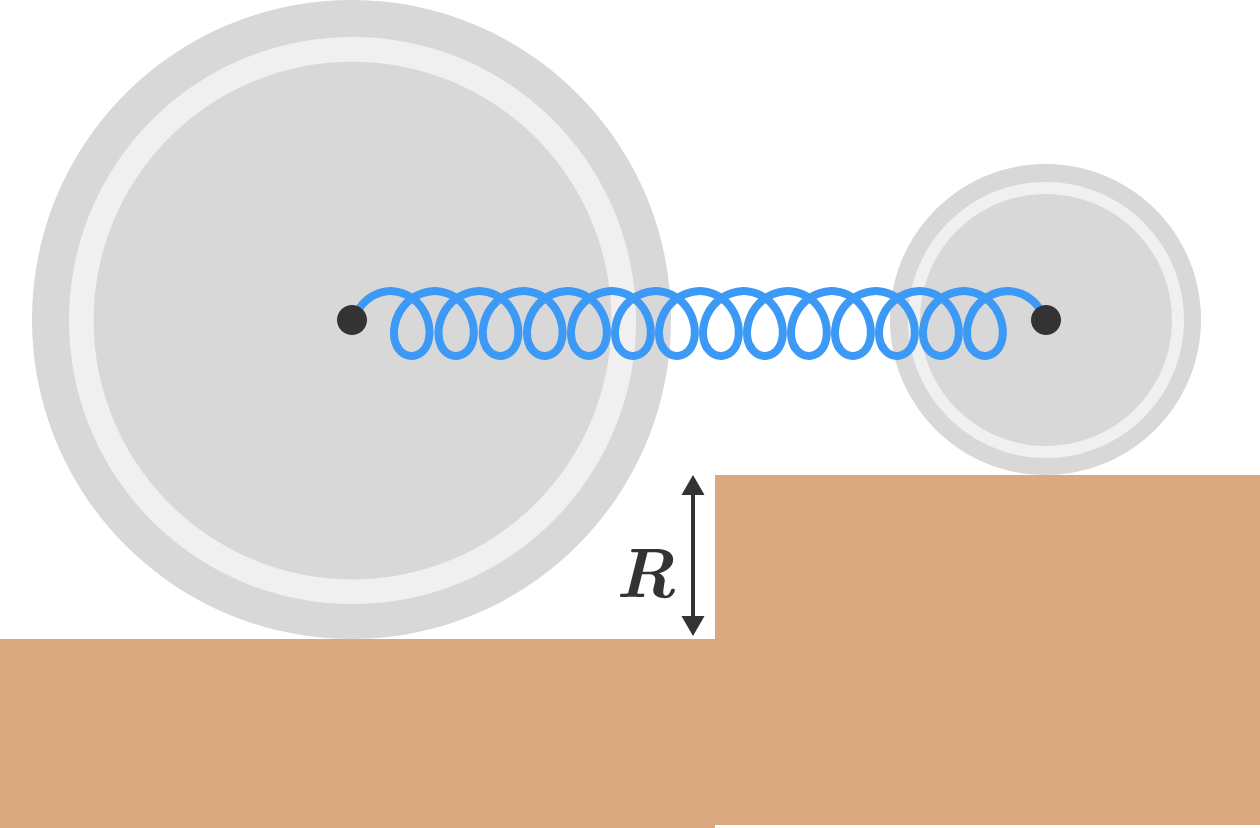# Oscillating disks on a stepTwo uniform circular disks of masses $M$ and $4M$ and radii $R$ and $2R,$ respectively, are connected by a massless spring of force constant $k$ and placed at different heights so that the spring is parallel to the horizontal, as shown above.

Initially, the spring is compressed, and then the system is released from rest. Assuming friction is large enough to prevent slipping, find the angular frequency of oscillation of the system.

If you answer comes in the form of $\sqrt{ \dfrac{ak}{bM}},$ where $a$ and $b$ are coprime positive integers, then enter $a+b$ as the final answer.

×